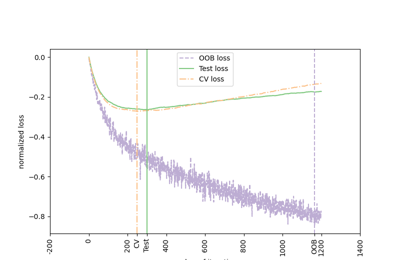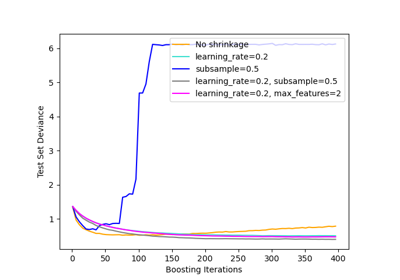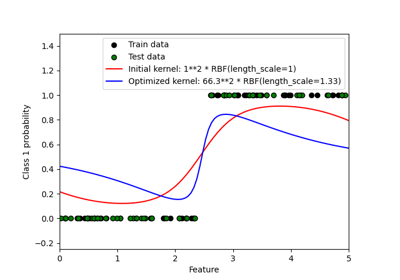# sklearn.metrics.log_loss¶

sklearn.metrics.log_loss(y_true, y_pred, *, eps='auto', normalize=True, sample_weight=None, labels=None)[source]

Log loss, aka logistic loss or cross-entropy loss.

This is the loss function used in (multinomial) logistic regression and extensions of it such as neural networks, defined as the negative log-likelihood of a logistic model that returns y_pred probabilities for its training data y_true. The log loss is only defined for two or more labels. For a single sample with true label $$y \in \{0,1\}$$ and a probability estimate $$p = \operatorname{Pr}(y = 1)$$, the log loss is:

$L_{\log}(y, p) = -(y \log (p) + (1 - y) \log (1 - p))$

Read more in the User Guide.

Parameters:
y_truearray-like or label indicator matrix

Ground truth (correct) labels for n_samples samples.

y_predarray-like of float, shape = (n_samples, n_classes) or (n_samples,)

Predicted probabilities, as returned by a classifier’s predict_proba method. If y_pred.shape = (n_samples,) the probabilities provided are assumed to be that of the positive class. The labels in y_pred are assumed to be ordered alphabetically, as done by LabelBinarizer.

epsfloat or “auto”, default=”auto”

Log loss is undefined for p=0 or p=1, so probabilities are clipped to max(eps, min(1 - eps, p)). The default will depend on the data type of y_pred and is set to np.finfo(y_pred.dtype).eps.

New in version 1.2.

Changed in version 1.2: The default value changed from 1e-15 to "auto" that is equivalent to np.finfo(y_pred.dtype).eps.

Deprecated since version 1.3: eps is deprecated in 1.3 and will be removed in 1.5.

normalizebool, default=True

If true, return the mean loss per sample. Otherwise, return the sum of the per-sample losses.

sample_weightarray-like of shape (n_samples,), default=None

Sample weights.

labelsarray-like, default=None

If not provided, labels will be inferred from y_true. If labels is None and y_pred has shape (n_samples,) the labels are assumed to be binary and are inferred from y_true.

New in version 0.18.

Returns:
lossfloat

Log loss, aka logistic loss or cross-entropy loss.

Notes

The logarithm used is the natural logarithm (base-e).

References

C.M. Bishop (2006). Pattern Recognition and Machine Learning. Springer, p. 209.

Examples

>>> from sklearn.metrics import log_loss
>>> log_loss(["spam", "ham", "ham", "spam"],
...          [[.1, .9], [.9, .1], [.8, .2], [.35, .65]])
0.21616...


## Examples using sklearn.metrics.log_loss¶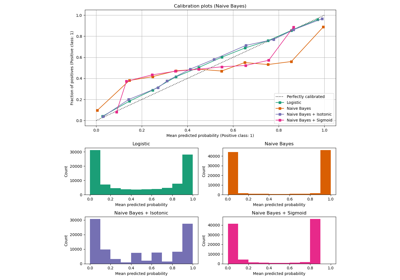Probability Calibration curves

Probability Calibration curves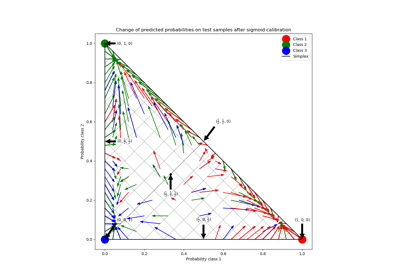Probability Calibration for 3-class classification

Probability Calibration for 3-class classification